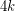Importance: High ✭✭✭
 Author(s): Jaeger, Francois
 Subject: Graph Theory » Coloring » » Nowhere-zero flows
 Keywords: nowhere-zero flow orientation
 Prize: none
 Posted by: mdevos on: March 7th, 2007
Conjecture   Every-edge-connected graph can be oriented so that(mod) for every vertex.

Jaeger called an orientation with the above property a modular-orientation, and observed that a graph has a modular-orientation if and only if it has a-flow. Thus, this conjecture may be seen as a sharp form of the 2+epsilon flow conjecture. For k=1, this problem is precisely the 3-flow conjecture, and for k=2, Jaeger showed that this conjecture (if true) would imply the 5-flow conjecture. If true, this conjecture would be best possible for every value of k.

The restriction of this conjecture to planar graphs is open, and has a dual formulation. See Mapping planar graphs to odd cycles.

Bibliography

[J] F. Jaeger, On circular flows in graphs. Finite and infinite sets, Vol. I, II (Eger, 1981), 391--402, Colloq. Math. Soc. János Bolyai, 37, North-Holland, Amsterdam, 1984.. MathSciNet

* indicates original appearance(s) of problem.